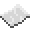# math.random

If neither min nor max were given, return a random floating point number between 0 and 1. If only min was given, return it; Otherwise, return a random integer between min and max.ExamplePrint 5 random numbers
Code
```<nowiki>
for i = 1, 5 do
print(math.random(1, 100))
end
</nowiki>
```
Output Five lines with (probably) different, random numbers.

math.random
Function
Syntax
math.random(
• min : number
• max : number
)

Returns number
API math
Source Lua (source)